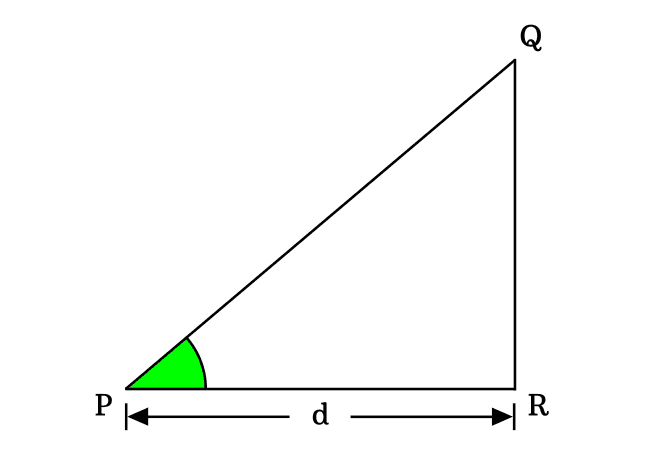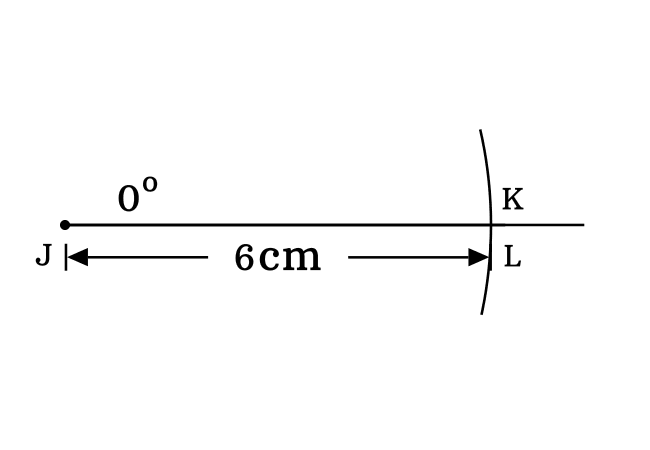# $\cot{(0^°)}$ Proof

According to trigonometric mathematics, the cot of zero degrees is equal to infinity.

$\cot{(0^°)} \,=\, \infty$

The cot of angle zero degrees can be derived in three different methods. One of them is trigonometric approach and remaining two are geometric methods. So, let’s learn every mathematical approach for proving that the cot of angle zero degrees is equal to infinity.

### Fundamental method

In this method, a geometric property between sides of a zero degree right triangle is considered for deriving the exact value of cotangent of angle zero degrees. Now, let’s study how to prove it by imagining a right triangle with zero degrees angle. The $\Delta RPQ$ is an example for a zero angle right triangle.According to the fundamental definition of cot function, it can be written in ratio of lengths of the sides. The cot function is written as $\cot{(0^°)}$ when the angle of a right triangle is zero degrees.

$\implies$ $\cot{(0^°)}$ $\,=\,$ $\dfrac{Length \, of \, Adjacent \, side}{Length \, of \, Opposite \, side}$

$\implies$ $\cot{(0^°)}$ $\,=\,$ $\dfrac{PR}{QR}$

In a zero degree right triangle, the length of opposite side is zero. Therefore $QR \,=\, 0$.

$\implies$ $\cot{(0^°)}$ $\,=\,$ $\dfrac{d}{0}$

$\,\,\,\therefore\,\,\,\,\,\,$ $\cot{(0^°)}$ $\,=\,$ $\infty$

### Experimental method

A right triangle with zero degrees angle is constructed in this method by the geometric tools for proving the cotangent of angle zero degrees practically. Let’s learn the procedure for constructing a right angled triangle with zero degrees angle.1. Consider a point $J$ and draw a horizontal line from this point by a ruler.
2. Now, take protractor and draw a zero degree line by coinciding the middle point with point $J$ and also coinciding its right side baseline with horizontal line. In fact, the zero degrees line is drawn over the horizontal straight line.
3. After that, set the distance between needle point and pencil lead point of a compass to any length (for example $6$ centimetres). Now, draw an arc on zero degrees line and it cuts the zero degrees line at point $K$.
4. Lastly, draw a perpendicular line to horizontal line from point $K$ by a set square. It intersects the horizontal line at point $L$. Thus, we construct a $\Delta KJL$. Remember that it is not possible to draw a perpendicular line to horizontal line from point $K$ because the points $K$ and $L$ are at same position in the plane.In $\Delta KJL$, the opposite side, adjacent side and hypotenuse are $\overline{KL}$, $\overline{JL}$ and $\overline{JK}$ respectively. $KL = 0\,cm$, $JK = 6\,cm$ and $JL = 6\,cm$. Now, we can calculate the cotangent of angle zero degrees by the ratio of lengths of its corresponding sides.

$\cot{(0^°)} \,=\, \dfrac{JL}{KL}$

$\implies$ $\cot{(0^°)} \,=\, \dfrac{6}{0}$

$\,\,\,\therefore\,\,\,\,\,\,$ $\cot{(0^°)} \,=\, \infty$

### Trigonometric method

In trigonometry, the cotangent of angle zero degrees can be proved from the fundamentals of trigonometry. According to quotient trigonometric identity, the cot function can be expressed in terms of cos and sine function.

$\cot{\theta} \,=\, \dfrac{\cos{\theta}}{\sin{\theta}}$

In this case, the angle $\theta$ is zero degrees.

$\implies$ $\cot{(0^°)} \,=\, \dfrac{\cos{(0^°)}}{\sin{(0^°)}}$

We know that the value of cos of zero degrees is one and the value of sine of zero degrees is equal to zero. Now, replace them in the above equation for evaluating the cot of $0$ degrees.

$\implies$ $\cot{(0^°)} \,=\, \dfrac{1}{0}$

$\,\,\,\therefore\,\,\,\,\,\,$ $\cot{(0^°)} \,=\, \infty$

These are the possible ways to prove that the co-tangent of angle zero degrees is equal to infinity in mathematics.

Latest Math Topics
Jun 26, 2023
Jun 23, 2023

###### Math Questions

The math problems with solutions to learn how to solve a problem.

Learn solutions

Practice now

###### Math Videos

The math videos tutorials with visual graphics to learn every concept.

Watch now

###### Subscribe us

Get the latest math updates from the Math Doubts by subscribing us.